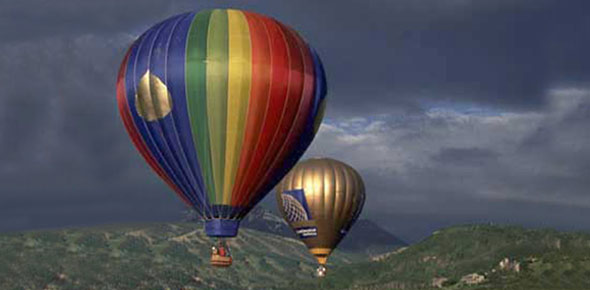# Chemistry: All The Gas Laws

10 Questions | Attempts: 1819
ShareSettingsBoyle's, Charles', Ideal Gas Law, Combined gas law.

• 1.
Which law compares gases at two different sets of conditions, with temperature as a constant and pressure and volume as variables?
• A.

Charle's Law

• B.

Boyle's Law

• C.

Combined Gas Law

• D.

Ideal gas law

• 2.
If pressure of a gas is 1.2 atmospheres with a volume of 12 L, how much pressure is exerted by the gas if the volume is changed to 9L? (Assume constant temperature)
• A.

.9 L

• B.

129.6 L

• C.

6.0 L

• D.

1.6 L

• 3.
If the pressure of a gas is 103.2 kPa at a temperature of 100 Celsius and a volume of 4L is changed to a lower pressure of 80 kPa and 100 degrees celsius, what will be the new volume? (Hint: kPa is kilo pascals -- a unit of measure for pressure).
• A.

2,064 L

• B.

0.3225 L

• C.

5.16 L

• D.

3.1 L

• 4.
A gas at a temperature of 80Celsius and a volume of 3.2 Liters is brought into an area with the temperature of 100 Celsius, what is the new volume if the pressure remains constant? Hint 1: This is Charles law (V1/T1 = V2/T2) Hint 2: ALL GAS PROBLEMS MUST use the KELVIN temperature scale. (To change from Celsius to Kelvin: add 273)
• A.

3.38 L

• B.

2.84 L

• C.

3.75 L

• D.

2.4 L

• 5.
A gas at a temperature of 34 Celsius and a volume of 6.3  Liters is brought into a new area where the gas expands to a volume of 8.0 Liters. What is the new temperature? (Assume constant Pressure)
• A.

43.17 K

• B.

389.84 K

• C.

0.164 K

• D.

1.48 K

• 6.
A gas in a balloon measures 2.2 L 1.1 atmospheres and 32 degrees Celsius. The environment is changed and the balloon is now 1.8 L and 1.4 atmospheres. What is the new temperature? Hint 1: Always change temperature to Kelvin scale. Hint 2: Pressure volume and temperature are all changed. Use the combined gas law:
• A.

317.6 K

• B.

33.32 K

• C.

384.3 K

• D.

0.02 K

• 7.
A gas in a balloon measures 3.6 L, 0.9 atmospheres and 34 degrees Celsius. The environment is changed and the balloon is now 1.4 atmospheres at a temperature of 38 degrees Celsius. What is the new volume?
• A.

2.59 L

• B.

4.6 L

• C.

220,961 L

• D.

2.34 L

• 8.
What is the volume of  2 moles of Helium at a temperature of 32 degreees celsius and a pressure of 1.1 atmospheres ? Given R = 0.08321 Latm/molK  and 8.314 kPa L/mol K Hint: There is only 1 set of conditions for this gas problem and the moles of the gas are given. This indicates the ideal gas law: PV = nRT.  Remember that is equal to the number of moles. P is the pressure, V is the volume and T is the temperature (change to Kelvin). The R MUST match the units in the rest of the problem.  IF pressure is given in atmosphere, then use R = 0.08321 Latm/molK . IF pressure was given in kPa, then use 8.314 Kpa L/mol K Note also that volume MUST be in Liters and Temperature (as for all gas problems) must be in Kelvin.
• A.

13.96 L

• B.

.45 L

• C.

46.14 L

• D.

4,610 L

• 9.
What is the temerature of  3.4 moles of Helium at a  pressure of 1.1 atmospheres and a volume of 3.7 Liters. (What is the temperature in Kelvin?) Given R = 0.08321 Latm/molK  and 8.314 kPa L/mol K
• A.

14.39 K

• B.

12.15 K

• C.

0.1 K

• D.

142 K

• 10.
How many moles is present in a container that is 79.9 Liters with a pressure of 105 kPa and a temperature of 24 degrees Celsius? Given R = 0.08321 Latm/molK  and 8.314 kPa L/mol K
• A.

2.7 moles

• B.

271 moles

• C.

3.4 moles

• D.

5.6 moles

## Related Topics

×

Wait!
Here's an interesting quiz for you.